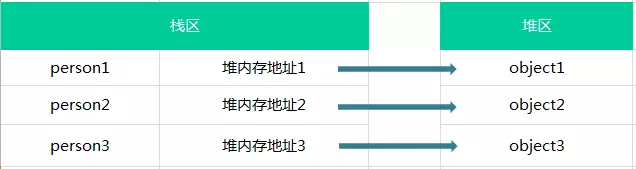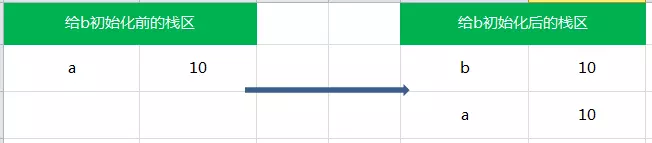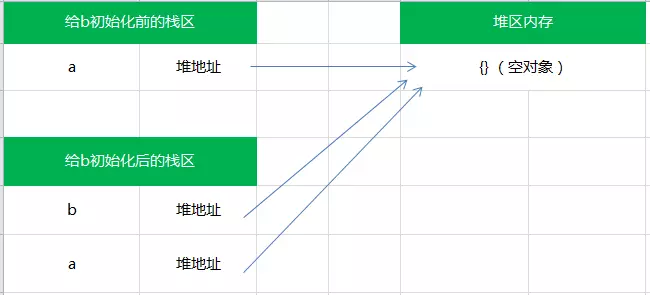# # 堆栈

## # 基本数据类型

1. 基本数据类型值不可变
``````let a = 'abc'
a = 'c'

console.log(a) // abc
``````
2. 基本类型的比较是值的比较
``````var a = 1;
var b = 1;
console.log(a === b);//true
``````

## # 引用类型``````var a = [1,2,3];
var b = [1,2,3];
console.log(a === b); // false
``````

## # 传值与传址的区别

``````var a = 10;
var b = a;

a ++;
console.log(a); // 11
console.log(b); // 10
````````````var a = {}; // a保存了一个空对象的实例
var b = a;  // a和b都指向了这个空对象

a.name = 'jozo';
console.log(a.name); // 'jozo'
console.log(b.name); // 'jozo'

b.age = 22;
console.log(b.age);// 22
console.log(a.age);// 22

console.log(a == b);// true
``````## # 深拷贝与浅拷贝

``````    // 内部方法：用户合并一个或多个对象到第一个对象
// 参数：
// target 目标对象  对象都合并到target里
// source 合并对象
// deep 是否执行深度合并
function extend(target, source, deep) {
for (key in source)
if (deep && (isPlainObject(source[key]) || isArray(source[key]))) {
// source[key] 是对象，而 target[key] 不是对象， 则 target[key] = {} 初始化一下，否则递归会出错的
if (isPlainObject(source[key]) && !isPlainObject(target[key]))
target[key] = {}

// source[key] 是数组，而 target[key] 不是数组，则 target[key] = [] 初始化一下，否则递归会出错的
if (isArray(source[key]) && !isArray(target[key]))
target[key] = []
// 执行递归
extend(target[key], source[key], deep)
}
// 不满足以上条件，说明 source[key] 是一般的值类型，直接赋值给 target 就是了
else if (source[key] !== undefined) target[key] = source[key]
}

// Copy all but undefined properties from one or more
// objects to the `target` object.
\$.extend = function(target){
var deep, args = slice.call(arguments, 1);

//第一个参数为boolean值时，表示是否深度合并
if (typeof target == 'boolean') {
deep = target;
//target取第二个参数
target = args.shift()
}
// 遍历后面的参数，都合并到target上
args.forEach(function(arg){ extend(target, arg, deep) })
return target
}
``````

\$.extend(true, target, [source, ...]) ⇒ target

Last Updated: 9/11/2019, 8:53:50 AM